Latest Banking jobs   »   Quantitative Aptitude Quiz For IBPS RRB...

# Quantitative Aptitude Quiz For IBPS RRB PO, Clerk Prelims 2021- 9th August

Directions (1-5): In each of the following questions two equations (I) and (II) are given, you have to solve both the equation and give answer.
(a) if x < y
(b) if x ≤ y
(c) if x > y
(d) if x ≥ y
(e) if x = y or no relationship can be obtained between x and y.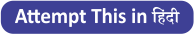Q1. (I) x² – 14x + 48 = 0
(II) y² – 18y + 80 = 0

Q2. (I) x³ + 328 = 2525
(II) y³ + 349 = 1680

Q3. (I) x² – 19x + 88 = 0
(II) y² – 21y + 108 = 0

Q4. (I) x³ = 1728
(II) y² = 144

Q5. (I) 2x² + 25x + 75 = 0
(II) 3y² + 26y + 56 = 0

Directions (6 – 10): In each of these questions, two equations (I) and (II) are given. You have to solve both the equations and answer the following questions.
(a) x > y
(b) x < y
(c) x ≥ y
(d) x ≤ y
(e) x = y or no relation

Q6. I. 2x² – 11x + 15 = 0
II. 2y² – 13y + 20 = 0

Q7. I. 30/x²=11/x-1
II. y² – 7y + 12 = 0

Q8. I. x² – 84 = 112
II. y³ + 1845 = 5220

Q9. I. x² + 18x + 80 = 0
II. y² + 14y + 48 = 0

Q10. I. 18x – 12y = 9
II. 16y – 20x = 2

Directions (11-15): In each of the following questions, two equations (I) and (II) are given, you have to solve both the equations and give answer.
(a) If x > y
(b) If x ≥ y
(c) If x < y
(d) If x ≤ y
(e) If x = y or no relation can be established between x and y.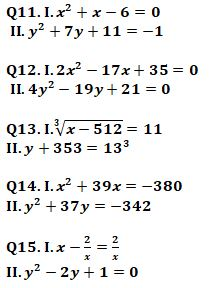Practice More Questions of Quantitative Aptitude for Competitive Exams:

###### Target IBPS RRB PO and Clerk Prelims 2021

Solutions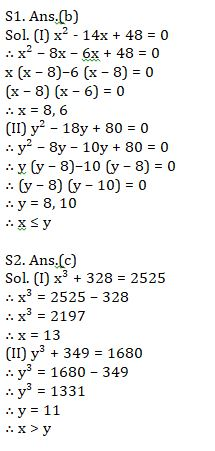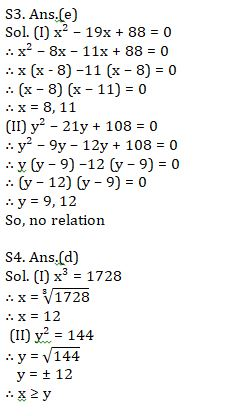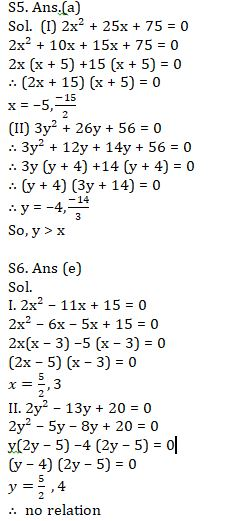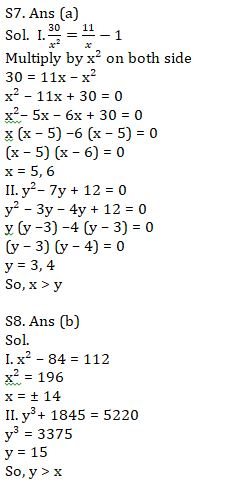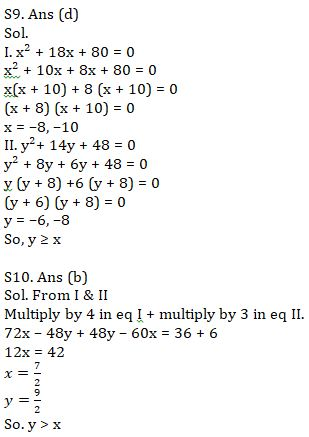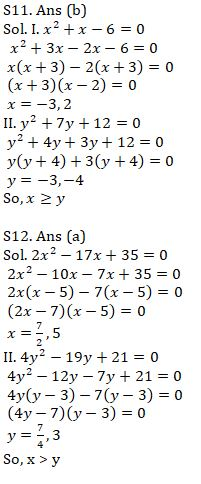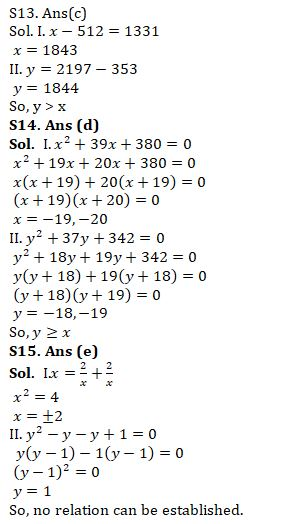#### Congratulations!Union Budget 2023-24: Free PDF